#35
164
11. 云栖社区>
2. 博客>
3. 正文

## 从能做什么到如何去做，一文带你快速掌握Python编程基础与实战

• Python能做什么?(常见应用场景介绍)
• 如何学习Python？
• Python语法基础实战
• Python面向对象编程实战
• 练熟基础：2048小游戏项目的实现与实战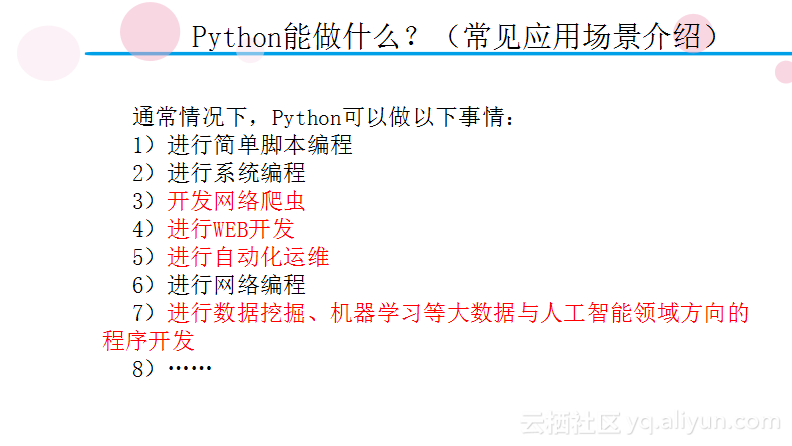Python基础知识入门

``````a1='abc'
a2="abc"
a3='''ab
c'''``````

``````a=1000
b=1
if(a>19 and a<30):
print(a)
if(b<9):
print(b)
elif(a>9 and a<=19):
print("a>9 and a<=19")
elif(a<9):
print("a<9")
else:
print("gsdajk")``````

``````a=0
while(a<10):
print("hello")
a+=1``````

``````a=["aa","b","c","d"]
for i in a:
print(i)``````
for进行常规循环的写法是for i in range()，也就是从X到Y的意思。比如for i in range(2,5)就是遍历2、3、4，这里值得注意的就是遍历不到最后的元素，在该例子中遍历不到5。如下列代码将会输出10次“hello”。
``````for i in range(0,10):
print("hello")``````

``````a=["aa","b","c","d"]
for i in a:
if(i=="c"):
break
print(i)``````

``````a=["aa","b","c","d"]
for i in a:
if(i=="c"):
continue
print(i)``````

``````for i in range(1,10):
for j in range(1,i+1):
print(str(i)+"*"+str(j)+"="+str(i*j),end="  ")
print()````````````for i in range(9,0,-1):
for j in range(i,0,-1):
print(str(i)+"*"+str(j)+"="+str(i*j),end="  ")
print()``````

``````i=10
def func():
print(i)
func()``````

``````def func():
i=10
print(i)
func()``````

``````i=10
def func():
global i
print(i)
func()``````

``````def 函数名(参数):
函数体``````

``````def abc():
print("abcde")
print("456")
abc()``````

``````def func2(a,b):
if(a>b):
print(str(a)+"比"+str(b)+"大")
else:
print(str(b)+"比"+str(a)+"大或者"+str(b)+"与"+str(a)+"相等")``````

``````import random
from time import sleep
from time import *
a=["中奖","不中奖","不中奖"]
print(random.choice(a))``````

random模块主要用于随机数的处理。上述代码使用random实现了一个抽奖功能，首先导入了random，然后建立一个列表，里面有“中奖”和“不中奖”选项，random.choice(a)将会随机在列表a中选择一个选项并输出对应的结果。time模块也有一些比较常用的功能，比如time.sleep()，其实现了延时功能。如果想要修改模块的源代码，只需要在lib目录下找到相应的模块进行修改即可。

``````fh=open("D:/我的教学/Python/20180120内训/第1天代码/文本1.txt","r",encoding="utf-8")
#文件读取

``````x=0
fh=open("D:/我的教学/Python/20180120内训/第1天代码/文本1.txt","r",encoding="gbk")
while True:
if(len(line2)==0):
break
print(line2)
x+=1
fh.close()``````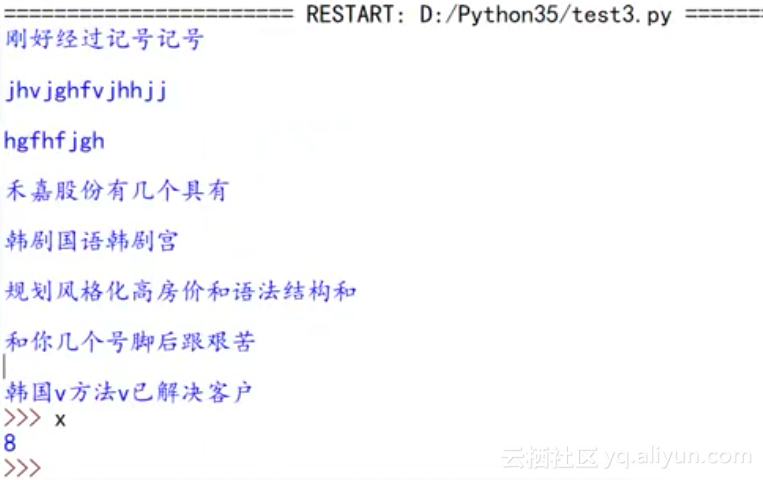``````data="一起学Python！"
fh2=open("D:/我的教学/Python/20180120内训/第1天代码/文本3.txt","w")
fh2.write(data)
fh2.close()``````

Python除了用于操作文件之外，还常用于操作数据库，本文以操作MySQL数据库为例讲解。在使用Python操作数据库之前需要先安装pymysql模块，在cmd命令行中输入“pip install pymysql”即可完成该模块的安装。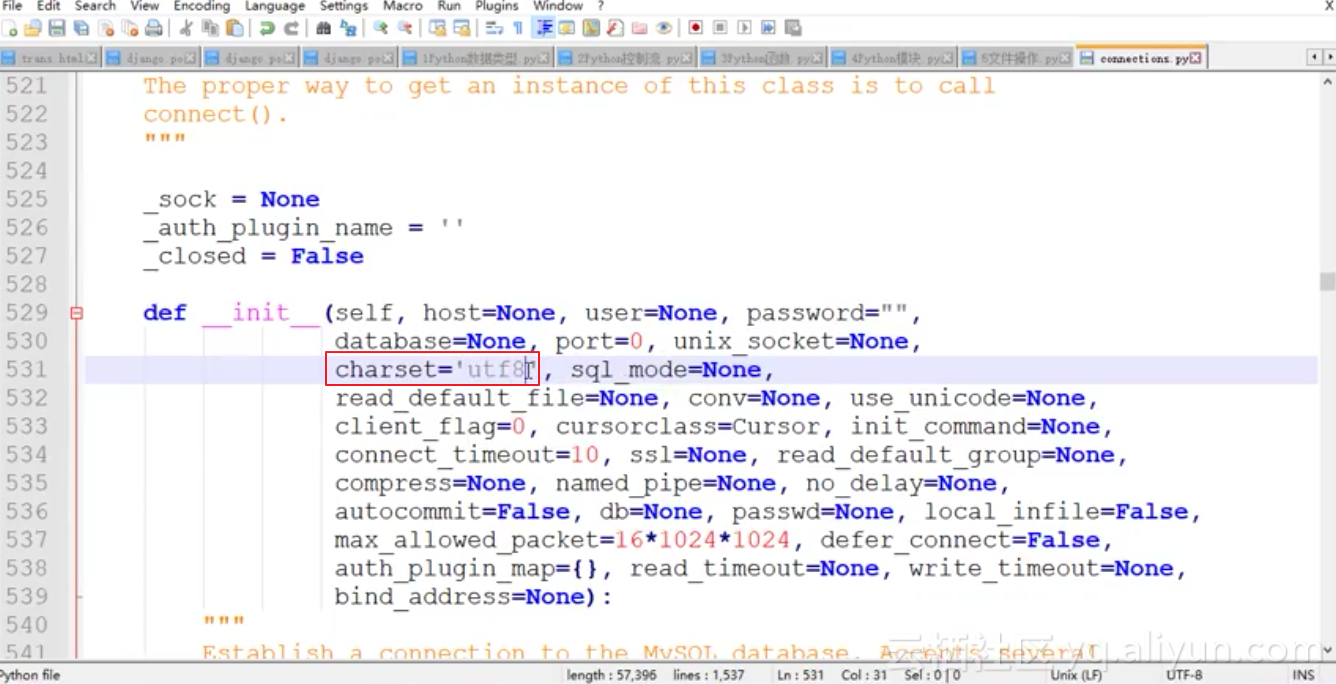``````import pymysql
conn=pymysql.connect(host="127.0.0.1",user="root",passwd="root",db="mypydb")
#执行sql语句-无返回
conn.query("INSERT INTO mytb(title,keywd) VALUES('new title','23456')")
conn.commit()
#执行SQL语句-有返回
cs=conn.cursor()
cs.execute("select * from mytb")
for i in cs:
print("当前是第"+str(cs.rownumber)+"行")
print("标题是："+i)
print("关键词是："+i)
conn.close()``````

``````try:
程序
except Exception as 异常名称:
异常处理部分``````
try后面的代码段就是尝试着去执行这段程序，如果出现异常则会触发except从句。except后面是对应的Exception类型，这里有一种的“必杀技”，就是直接使用Exception——通用异常，只要发生异常，Exception都会将其捕获到。as表示对于异常起一个别名，而接下来就是对应的异常处理的代码。
``````try:
for i in range(0,10):
print(i)
if(i==4):
print(jkj)
print("hello")
except Exception as err:
print(err)``````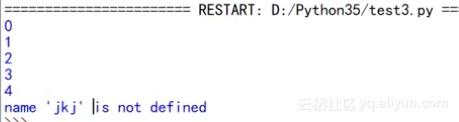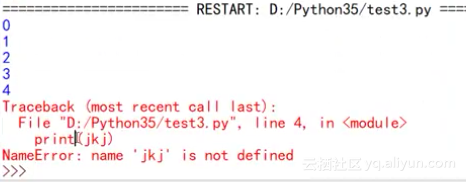``````for i in range(0,10):
try:
print(i)
if(i==4):
print(jkj)
except Exception as err:
print(err)
print("hello")``````

``````class 类名:
类里面的内容``````

``````class cl1:
pass``````

``````class cl2:
def __init__(self):
print("I am cl2 self!")``````

``````class cl3:
def __init__(self,name,job):
print("My name is "+name+" My job is "+job)``````

``````class cl4:
def __init__(self,name,job):
self.myname=name
self.myjob=job``````

``````class cl5:
def myfunc1(self,name):
print("hello "+name)

class cl6:
def __init__(self,name):
self.myname=name
def myfunc1(self):
print("hello "+self.myname)``````

``````class father():
def speak(self):
print("I can speak!")``````

``````class son(father):
pass``````

``````class mother():
def write(self):
print("I can write")``````

``````class daughter(father,mother):
def listen(self):
print("I can listen!")``````

``````class son2(father):
def speak(self):
print("I can speak 2!")``````

Python数据挖掘与机器学习技术入门实战
Python数据挖掘与机器学习，快速掌握聚类算法和关联分析

+ 关注